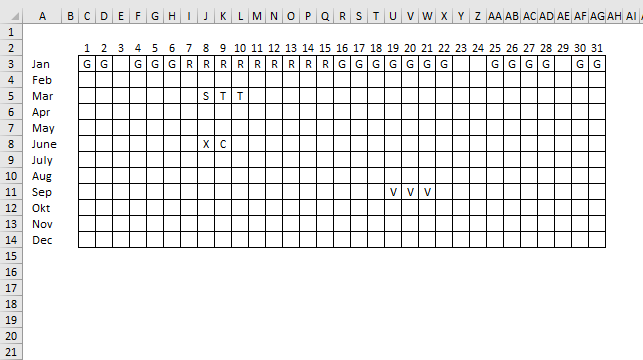Author: Oscar Cronquist Article last updated on December 30, 2018One more question for the Calendar that you have set up above can we have a excel formula which will give us a below table
StarWk EndWk Name
1 2 G
4 6 G
7 15 R ... and so on

Question found here.

The image above shows three different formulas that extract groups based on adjacent text names from a calendar, the calendar is shown in the top image. The only exception is a range that spans over at least two months, it will be divided into two date ranges.

Array Formula in cell B3:

=SMALL(IF((Sheet1!\$C\$3:\$AG\$14<>Sheet1!\$B\$3:\$AF\$14)* (Sheet1!\$C\$3:\$AG\$14<>""), DATE(2018, ROW(\$1:\$12), Sheet1!\$C\$2:\$AG\$2), ""), ROWS(\$A\$1:A1))

To enter an array formula, type the formula in a cell then press and hold CTRL + SHIFT simultaneously, now press Enter once. Release all keys.

The formula bar now shows the formula with a beginning and ending curly bracket telling you that you entered the formula successfully. Don't enter the curly brackets yourself.

Copy cell A2 and  paste it down as far as needed.

### Explaining formula in cell B3

#### Step 1 - Compare cell ranges

The less than and greater than signs combined returns TRUE if a cell is not equal to the next.

Sheet1!\$C\$3:\$AG\$14<>Sheet1!\$B\$3:\$AF\$14

becomes

{"G", "G", 0, ... I made the array shorter ... , 0}<>{0, "G", "G", ... , 0}

and returns

{TRUE, FALSE, TRUE, ...  , FALSE}

#### Step 2 - Check if cell is not empty

Sheet1!\$C\$3:\$AG\$14<>""

returns

{TRUE, TRUE, FALSE, ... , FALSE}

#### Step 3 - Multiply arrays

(Sheet1!\$C\$3:\$AG\$14<>Sheet1!\$B\$3:\$AF\$14)* (Sheet1!\$C\$3:\$AG\$14<>"")

becomes

{TRUE, FALSE, TRUE,  ... , FALSE}* {TRUE, TRUE, FALSE,  ... , FALSE}

and returns

{1,0,0,... ,0}

#### Step 4 - Convert array into dates

The IF function has three arguments, the first one must be a logical expression. If the expression evaluates to TRUE then one thing happens (argument 2) and if FALSE another thing happens (argument 3). The following lines explain the logical expression:

IF((Sheet1!\$C\$3:\$AG\$14<>Sheet1!\$B\$3:\$AF\$14)* (Sheet1!\$C\$3:\$AG\$14<>""), DATE(2018, ROW(\$1:\$12), Sheet1!\$C\$2:\$AG\$2), "")

becomes

IF({1,0,0,... ,0}, DATE(2018, ROW(\$1:\$12), Sheet1!\$C\$2:\$AG\$2), "")

becomes

IF({1,0,0,... ,0}, DATE(2018, {1;2;3;4;5;6;7;8;9;10;11;12}, Sheet1!\$C\$2:\$AG\$2), "")

and returns

{43101,"","", ... ,""}

#### Step 5 - Extract dates

To be able to return a new value in a cell each I use the SMALL function to filter column numbers from smallest to largest.

The ROWS function keeps track of the numbers based on an expanding cell reference. It will expand as the formula is copied to the cells below.

SMALL(IF((Sheet1!\$C\$3:\$AG\$14<>Sheet1!\$B\$3:\$AF\$14)* (Sheet1!\$C\$3:\$AG\$14<>""), DATE(2018, ROW(\$1:\$12), Sheet1!\$C\$2:\$AG\$2), ""), ROWS(\$A\$1:A1))

becomes

SMALL({43101,"","", ... ,""}, ROWS(\$A\$1:A1))

becomes

SMALL({43101,"","", ... ,""}, 1)

and returns 43101 (1/1/2018) in cell B3.

Array Formula in cell C3:

=SMALL(IF((Sheet1!\$C\$3:\$AG\$14<>Sheet1!\$D\$3:\$AH\$14)* (Sheet1!\$C\$3:\$AG\$14<>""), DATE(2018, ROW(\$1:\$12), Sheet1!\$C\$2:\$AG\$2), ""), ROWS(\$A\$1:A1))

Copy cell B2 and  paste it down as far as needed. I am not going to explain this formula, it is very similar to the one in cell B3.

Formula in cell D3:

=INDEX(Sheet1!\$C\$3:\$AG\$14,MONTH(B3),DAY(B3))

Copy cell C2 and  paste it down as far as needed.

### Explaining formula in cell D3

#### Step 1 - Calculate month number from 1 to 12 based on date

The MONTH function extracts the month as a number from an Excel date.

MONTH(B3)

becomes

MONTH(1/1/2018)

becomes

MONTH(43101)

and returns 1.

#### Step 2 - Calculate day number based on date

The DAY function extracts the day as a number from an Excel date.

DAY(B3)

becomes

DAY(1/1/2018)

becomes

DAY(43101)

and returns 1.

#### Step 3 - Get text name based on month and day

The INDEX function returns a value based on a cell reference and column/row numbers.

INDEX(Sheet1!\$C\$3:\$AG\$14,MONTH(B3),DAY(B3))

becomes

INDEX(Sheet1!\$C\$3:\$AG\$14,1,1)

and returns "G" in cell D3.

### Get the Excel fileExtract-dates-and-name-from-block-schedule.xlsx